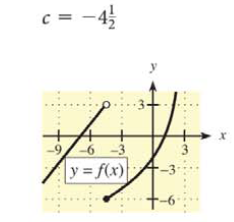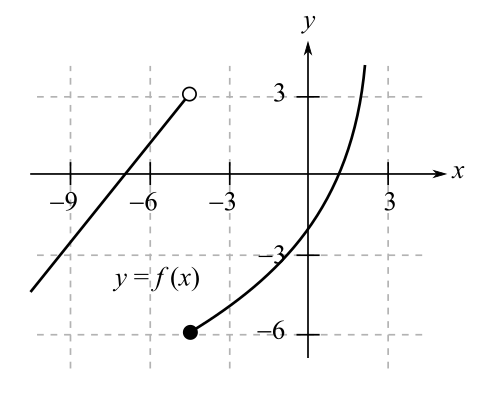Chapter 9.1, Problem 9E### Mathematical Applications for the ...

11th Edition
Ronald J. Harshbarger + 1 other
ISBN: 9781305108042

#### Solutions

Chapter
Section### Mathematical Applications for the ...

11th Edition
Ronald J. Harshbarger + 1 other
ISBN: 9781305108042
Textbook Problem

# In Problems 7-10, use the graph of y = f ( x ) and the given c-value to find the following, whenever they exist.(a) lim x → c f ( x ) (b) lim x → c f ( x ) (c) lim x → c f ( x ) (d)   f ( c ) c = −   4 1 2(a)

To determine

The value of limxcf(x) where c=412 using the graph,Explanation

Given Information:

The value of c is 412 and the graph of y=f(x) is

Explanation:

Consider the provided graph,

The provided value of c is 412.

That is, c=4.5.

To find limx4

(b)

To determine

The value of limxc+f(x) where c=412 using the graph,(c)

To determine

The value of limxcf(x) where c=412 using the graph,(d)

To determine

The value f(c) where c=412 using the graph,### Still sussing out bartleby?

Check out a sample textbook solution.

See a sample solution

#### The Solution to Your Study Problems

Bartleby provides explanations to thousands of textbook problems written by our experts, many with advanced degrees!

Get Started

#### Solve the equations in Exercises 126. (x+1)(x+2)2+(x+1)2(x+2)=0

Finite Mathematics and Applied Calculus (MindTap Course List)

#### Subtract the following numbers. 23. $127 less$33

Contemporary Mathematics for Business & Consumers

#### True or False: converges.

Study Guide for Stewart's Multivariable Calculus, 8th

#### Draw a scatterplot for which r = 1.

Introduction To Statistics And Data Analysis

#### For , an upper bound estimate for s − s6 (where s6 is the sixth the series does not converge

Study Guide for Stewart's Single Variable Calculus: Early Transcendentals, 8th